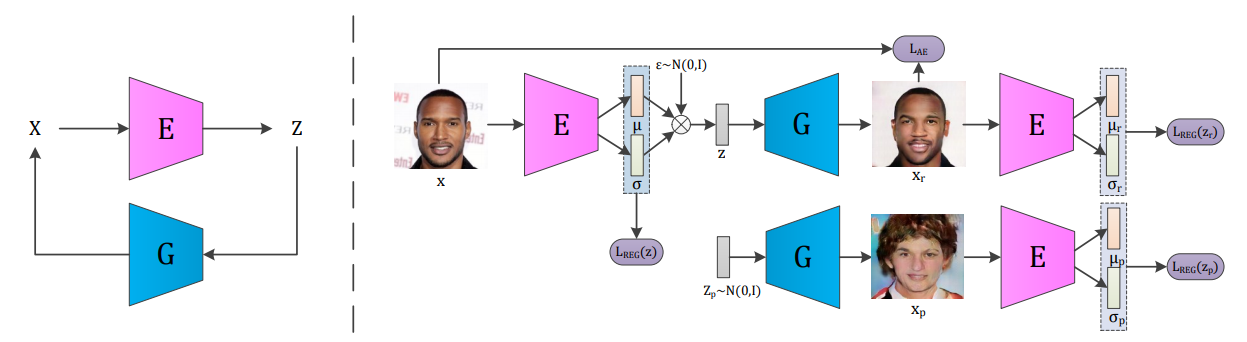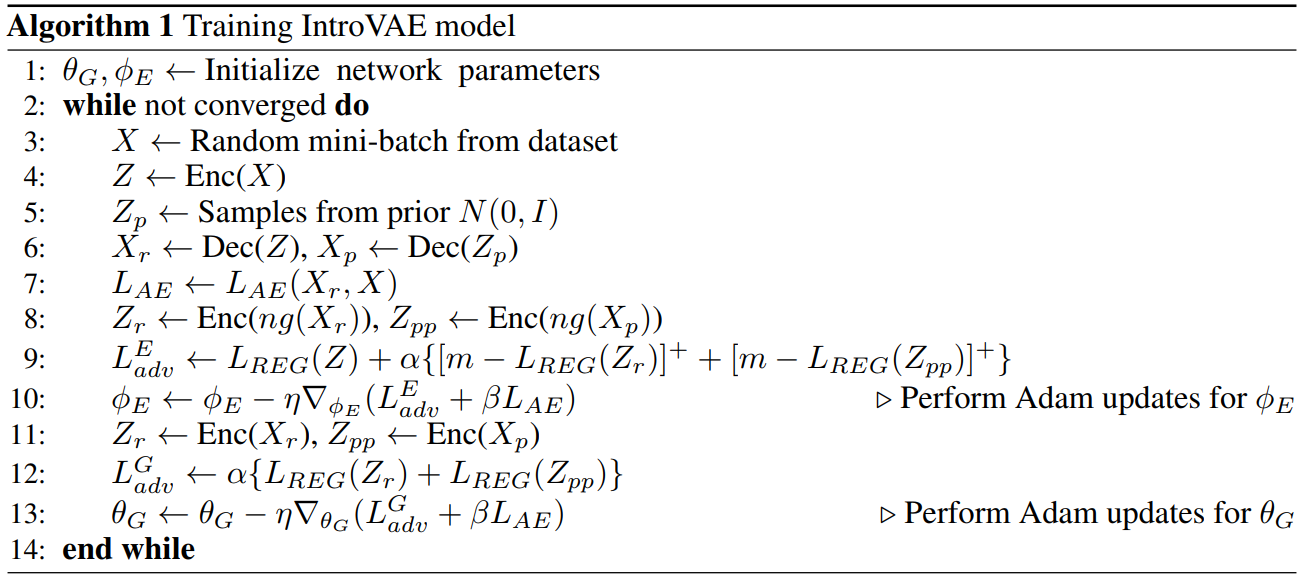# IntroVAE: Introspective Variational Autoencoders for Photographic Image Synthesis

## WHY?

VAE can learn useful representation while GAN can sample sharp images.

## WHAT?

Introspective Variational Autoencoder(IVAE) combines the advantage of VAE and GAN to make a model to learn useful representation and output sharp images. IVAE uses encoder to introspectively estimate the generated samples and the training data as a discriminator.L_{AE} = -E_{q_{\phi}(z|x)}log p_{\theta}(x|z) = \frac{1}{2}\sum_{i=1}^N\|x_{r,i}-x_i\|^2_F\\
L_{REG} = D_{KL}(q_{\phi}(z|x)\|p(z)) = \frac{1}{2}\sum_{i=1}^N(1+log(\sigma_i^2) - \mu_i^2 - \sigma_i^2)\\
L_E(x,z) = E(x) + [m-E(G(z))]^+ + L_{AE}(x) = L_{REG}(Enc(x)) + \alpha\sum_{s=r,p}[m-L_{REG}(Enc(ng(x_s)))]^+ + \beta L_{AE}(x, x_r)\\
L_G(z) = E(G(z)) + L_{AE}(x) = \alpha \sum_{s=r,p} L_{REG}(Enc(x_s)) + \beta L_{AE}(x)

Algorithm is as follows.## So?

IVAE achieved realistic quality reconstruction and sample from CelebA, CelebA-HQ, and LSUN Bedroom. Also the representations learned from IVAE showed meaningful latent manifold.

## Critic

Amazing image quality!

Powered by aiden